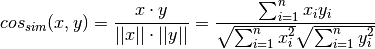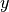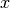Shortcuts

# Cosine Similarity¶

## Module Interface¶

class torchmetrics.CosineSimilarity(reduction='sum', **kwargs)[source]

Computes the Cosine Similarity between targets and predictions:whereis a tensor of target values, andis a tensor of predictions.

As input to forward and update the metric accepts the following input:

• preds (Tensor): Predicted float tensor with shape (N,d)

• target (Tensor): Ground truth float tensor with shape (N,d)

As output of forward and compute the metric returns the following output:

Parameters

Example

>>> from torchmetrics import CosineSimilarity
>>> target = torch.tensor([[0, 1], [1, 1]])
>>> preds = torch.tensor([[0, 1], [0, 1]])
>>> cosine_similarity = CosineSimilarity(reduction = 'mean')
>>> cosine_similarity(preds, target)
tensor(0.8536)


Initializes internal Module state, shared by both nn.Module and ScriptModule.

## Functional Interface¶

torchmetrics.functional.cosine_similarity(preds, target, reduction='sum')[source]

Computes the Cosine Similarity between targets and predictions:whereis a tensor of target values, andis a tensor of predictions.

Parameters

Example

>>> from torchmetrics.functional.regression import cosine_similarity
>>> target = torch.tensor([[1, 2, 3, 4],
...                        [1, 2, 3, 4]])
>>> preds = torch.tensor([[1, 2, 3, 4],
...                       [-1, -2, -3, -4]])
>>> cosine_similarity(preds, target, 'none')
tensor([ 1.0000, -1.0000])

Return type

Tensor

© Copyright Copyright (c) 2020-2023, Lightning-AI et al... Revision 6853c553.

Built with Sphinx using a theme provided by Read the Docs.
Versions
latest
stable
v0.11.2
v0.11.1
v0.11.0
v0.10.3
v0.10.2
v0.10.1
v0.10.0
v0.9.3
v0.9.2
v0.9.1
v0.9.0
v0.8.2
v0.8.1
v0.8.0
v0.7.3
v0.7.2
v0.7.1
v0.7.0
v0.6.2
v0.6.1
v0.6.0
v0.5.1
v0.5.0
v0.4.1
v0.4.0
v0.3.2
v0.3.1
v0.3.0
v0.2.0
v0.1.0